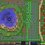# IIT JEE 1982 Mathematics: The actual Subjective Questions

Order has not been preserved. Before trying this, you may want to solve IIT JEE 1982 Mathematics Archives.

• Q1 Let $f$ be a one-one function with domain {x, y, z} and range {1, 2, 3}. It is given that exactly one of the following statements is true and the remaining two are false: $f(x)=1$, $f(y)≠1$, $f(z)≠2$. Then determine $f^{-1}(1)$.

• Q2 Find the equation of the circle passing through the point $(-4, 3)$ and touching the lines $x+y=2$ and $x-y=2$.

• Q3 Show that the equation $e^{\sin x}-e^{-\sin x}-4=0$ has no solution.

• Q4 Find the co-ordinates of the points of intersection of the curves $y=\cos x$ and $y=\sin3x$, if $-\frac \pi 2 ≤x ≤ \frac \pi 2$.

• Q5 A is a point on the parabola $y^2=4ax$. The Normal at A cuts the parabola again at point B. If AB subtends a right angle at the vertex of the parabola, then find the slope of AB.

• Q6 Use the formula $\displaystyle \lim_{a\to 0} \frac{a^x-1}{x}=\ln a$ to find $\displaystyle \lim_{x\to 0}\frac {2^x-1}{(1+x)^{1/2}-1}$ .

• Q7 Determine the values of $a, b, c$ for which the function $f(x)$ is continuous at $x=0$. $f(x)=\begin{cases} \Large \frac{\sin[a(x+1)]+\sin x}{x}, \normalsize x<0 \\ c, x=0\\ \Large \frac{(x+bx^2)^{1/2}-x^{1/2}}{bx^{3/2}}, \normalsize x>0 \end{cases}$

• Q8 Let $f$ be a twice differentiable function, such that $f''(x)=-f(x)$, $f'(x)=g(x)$, $h(x)=[f(x)]^2+[g(x)]^2$, $h(5)=11$. Find $h(10)$ if $h(5)=11$.

• Q9 $mn$ squares of equal size are arranged to form a rectangle of dimension $m$ by $n$, where $m, n \in\mathbb N$. Two squares will be called "neighbours" if they have exactly one common side. A number is written in each square such that the number in any square is the arithmetic mean of the numbers written in neighbouring squares. Show that this is possible only if all the numbers used are equal.

• Q10 If $a_1$ $a_2$, $...$, $a_n$ are in arithmetic progression, where $a_i>0$ for all $i$, show that $\frac{1}{a_1+\sqrt{a_2}}+\frac{1}{\sqrt{a_2}+\sqrt{a_3}}+\frac{1}{\sqrt{a_3}+\sqrt{a_4}}+...+\frac{1}{\sqrt{a_{n-1}}+\sqrt{a_n}}=\frac{n-1}{\sqrt{a_1}+\sqrt{a_n}}$

• Q11 Without expanding a determinant at any stage, show that $\begin{vmatrix} x^2+x & x+1 & x-2\\ 2x^2+3x-1 & 3x & 3x-3\\ x^2+2x+3 & 2x-1 & 2x-1\\ \end{vmatrix} = xA+B$where A and B are determinants of order 3, not involving $x$.

• Q12 For any real $t$, $x=\frac12(e^t+e^{-t}), \ y=\frac12(e^t-e^{-t})$ is a point on the hyperbola $x^2-y^2=1$. Show that the area bounded by the hyperbola and the lines joining the centre to the points corresponding to $t_1$ and $-t_1$ is $t_1$.

• Q13 If f(x) and g(x) are differentiable functions for $0≤x≤1$ such that $f(0)=2$, $g(0)=0$, $f(1)=6$, $g(1)=2$, then show that there exists $c$ satisfying $0 and $f'(c)=2g'(c)$.

• Q14 Does there exist a geometric progression containing 27, 8 and 12 as three of its terms? If it exists, how many such progressions are possible?

• Q15 A and B are two candidates seeking admission in IIT. The probability that A is selected is 0.5 and the probability that A and B are selected is at most 0.3. Then is it possible that the probability of B getting selected is 0.9?

• Q16 Find the shortest distance between the point $(0, c)$ from the parabola $y=x^2$ , where $0≤c≤1$.

• Q17 If $\large ax^2+\frac{b}{x}≥c \ \forall x \in R^+$ where $a>0$ and $b>0$, then show that $27ab^2≥4c^3$

• Q18 Show that $\large \int_0^{\pi}xf(\sin x) \ dx=\frac \pi 2 \int_0^{\pi}f(\sin x)\ dx$.

• Q19 Find the value of $\large \int_{-1}^\frac 32 |x \sin (\pi x)| \ dx$.

Note Do not post the solution to these problems in the comments. Instead consider, if you want to, posting them under the respective problem. If you can tell the marks of any of these questions, do inform.Note by Shubhamkar Ayare
4 years, 5 months ago

This discussion board is a place to discuss our Daily Challenges and the math and science related to those challenges. Explanations are more than just a solution — they should explain the steps and thinking strategies that you used to obtain the solution. Comments should further the discussion of math and science.

When posting on Brilliant:

• Use the emojis to react to an explanation, whether you're congratulating a job well done , or just really confused .
• Ask specific questions about the challenge or the steps in somebody's explanation. Well-posed questions can add a lot to the discussion, but posting "I don't understand!" doesn't help anyone.
• Try to contribute something new to the discussion, whether it is an extension, generalization or other idea related to the challenge.
• Stay on topic — we're all here to learn more about math and science, not to hear about your favorite get-rich-quick scheme or current world events.

MarkdownAppears as
*italics* or _italics_ italics
**bold** or __bold__ bold
- bulleted- list
• bulleted
• list
1. numbered2. list
1. numbered
2. list
Note: you must add a full line of space before and after lists for them to show up correctly
paragraph 1paragraph 2

paragraph 1

paragraph 2

[example link](https://brilliant.org)example link
> This is a quote
This is a quote
    # I indented these lines
# 4 spaces, and now they show
# up as a code block.

print "hello world"
# I indented these lines
# 4 spaces, and now they show
# up as a code block.

print "hello world"
MathAppears as
Remember to wrap math in $$ ... $$ or $ ... $ to ensure proper formatting.
2 \times 3 $2 \times 3$
2^{34} $2^{34}$
a_{i-1} $a_{i-1}$
\frac{2}{3} $\frac{2}{3}$
\sqrt{2} $\sqrt{2}$
\sum_{i=1}^3 $\sum_{i=1}^3$
\sin \theta $\sin \theta$
\boxed{123} $\boxed{123}$

## Comments

There are no comments in this discussion.

×

Problem Loading...

Note Loading...

Set Loading...